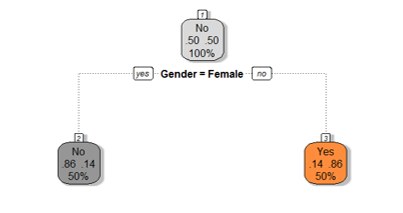• No products in the cart.

# 204.3.10 Pruning a Decision Tree in Python

Link to the previous post: https://statinfer.com/204-3-9-the-problem-of-overfitting-the-decision-tree

## Pruning

• Growing the tree beyond a certain level of complexity leads to overfitting.
• In our data, age doesn’t have any impact on the target variable.
• Growing the tree beyond Gender is not going to add any value. Need to cut it at Gender.
• This process of trimming trees is called Pruning.### Pruning to Avoid Overfitting

• Pruning helps us to avoid overfitting
• Generally it is preferred to have a simple model, it avoids overfitting issue
• Any additional split that does not add significant value is not worth while.
• We can avoid overfitting by changing the parameters like
• max_leaf_nodes
• min_samples_leaf
• max_depth
• Pruning Parameters
• max_leaf_nodes
• Reduce the number of leaf nodes
• min_samples_leaf
• Restrict the size of sample leaf
• Minimum sample size in terminal nodes can be fixed to 30, 100, 300 or 5% of total
• max_depth
• Reduce the depth of the tree to build a generalized tree
• Set the depth of the tree to 3, 5, 10 depending after verification on test data

### Code-Tree Pruning

```#We will rebuild a new tree by using above data and see how it works by tweeking the parameteres

dtree = tree.DecisionTreeClassifier(criterion = "gini", splitter = 'random', max_leaf_nodes = 10, min_samples_leaf = 5, max_depth= 5)
dtree.fit(X_train,y_train)
```
```DecisionTreeClassifier(class_weight=None, criterion='gini', max_depth=5,
max_features=None, max_leaf_nodes=10, min_samples_leaf=5,
min_samples_split=2, min_weight_fraction_leaf=0.0,
presort=False, random_state=None, splitter='random')```
```predict3 = dtree.predict(X_train)
print(predict3)
```
```[1 1 0 0 0 1 1 1 1 0 0 1 0 0]
```
```predict4 = dtree.predict(X_test)
print(predict4)
```
```[1 1 0 0 0 1]
```
```#Accuracy of the model that we created with modified model parameters.
score2 = dtree.score(X_test, y_test)
score2
```
`0.83333333333333337/`

The next post is a practice session on tree building model selection.

Link to the next post : https://statinfer.com/204-3-11-practice-tree-building-model-selection

0 responses on "204.3.10 Pruning a Decision Tree in Python"